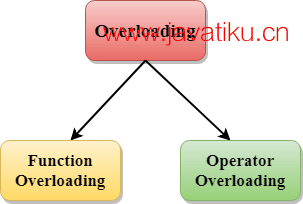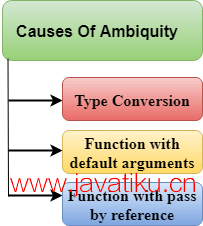# C++教程-C++重载（函数和运算符）## C++重载（函数和运算符）

• 方法
• 构造函数和
• 索引属性

### C++中的重载类型有：

• 函数重载
• 运算符重载### C++函数重载示例

// 当参数数量不同时的函数重载示例代码。

``````#include <iostream>
using namespace std;
class Cal {
public:
static int add(int a, int b) {
return a + b;
}
static int add(int a, int b, int c) {
return a + b + c;
}
};
int main(void) {
Cal C; // 类对象声明。
cout << C.add(10, 20) << endl;
return 0;
}``````

``30 55 ``

// 带有不同类型参数的函数重载示例程序。

``````#include<iostream>
using namespace std;
int mul(int, int);
float mul(float, int);

int mul(int a, int b)
{
return a * b;
}
float mul(double x, int y)
{
return x * y;
}
int main()
{
int r1 = mul(6, 7);
float r2 = mul(0.2, 3);
std::cout << "r1 is : " << r1 << std::endl;
std::cout << "r2 is : " << r2 << std::endl;
return 0;
}``````

``````r1 is : 42
r2 is : 0.6 ``````

### 函数重载和二义性

• 类型转换。
• 带有默认参数的函数。
• 带有按引用传递的函数。• 类型转换：

``````#include<iostream>
using namespace std;
void fun(int);
void fun(float);
void fun(int i)
{
std::cout << "i的值为：" << i << std::endl;
}
void fun(float j)
{
std::cout << "j的值为：" << j << std::endl;
}
int main()
{
fun(12);
fun(1.2);
return 0;
}``````

• 带有默认参数的函数

``````#include<iostream>
using namespace std;
void fun(int);
void fun(int, int);
void fun(int i)
{
std::cout << "i的值为：" << i << std::endl;
}
void fun(int a, int b = 9)
{
std::cout << "a的值为：" << a << std::endl;
std::cout << "b的值为：" << b << std::endl;
}
int main()
{
fun(12);
return 0;
}``````

• 带有引用传递的函数

``````#include <iostream>
using namespace std;
void fun(int);
void fun(int &);
int main()
{
int a = 10;
fun(a); // 错误，哪个f()？
return 0;
}
void fun(int x)
{
std::cout << "x的值为：" << x << std::endl;
}
void fun(int &b)
{
std::cout << "b的值为：" << b << std::endl;
}``````

### C++运算符重载

• Sizeof
• 成员选择符(.)
• 成员指针选择符(*)
• 三元运算符(?:)

``````return_type class_name : : operator op(argument_list)
{
// 函数体
}``````

class_name是类的名称。

operator op是运算符函数，其中op是要重载的运算符，operator是关键字。

### 运算符重载的规则

• 只能重载现有的运算符，不能重载新的运算符。
• 重载的运算符至少包含一个用户自定义数据类型的操作数。
• 不能使用友元函数重载某些运算符，但可以使用成员函数重载这些运算符。
• 当一元运算符通过成员函数重载时，不需要显式参数，但如果通过友元函数重载，则需要一个参数。
• 当二元运算符通过成员函数重载时，需要一个显式参数，如果通过友元函数重载，则需要两个显式参数。

### C++运算符重载示例

// 重载一元运算符++的程序。

``````#include <iostream>
using namespace std;
class Test
{
private:
int num;
public:
Test(): num(8){}
void operator++() {
num = num+2;
}
void Print() {
cout<<"Count 的值为: "<<num;
}
};
int main()
{
Test tt;
++tt; // 调用函数 "void operator++()"
tt.Print();
return 0;
}``````

``Count 的值为: 10 ``

// 重载二元运算符的程序。

``````#include <iostream>
using namespace std;
class A
{
int x;
public:
A(){}
A(int i)
{
x=i;
}
void operator+(A);
void display();
};

void A::operator+(A a)
{
cCopy code
int m = x+a.x;
cout<<"两个对象相加的结果为："<<m;
}
int main()
{
A a1(5);
A a2(4);
a1+a2;
return 0;
}``````

``两个对象相加的结果为：9``